Articles Members Online:
 -Article/Tip Search -News Group-Delphi/Pascal -CBuilder/C++ -C#Builder/C# -JBuilder/Java -Kylix Member Area -Home -Account Center -Top 10 NEW!! -Submit Article/Tip -Forums Upgraded!! -My Articles -Edit Information -Login/Logout -Become a Member -Why sign up! -Newsletter -Chat Online! -Indexes NEW!! Employment -Build your resume -Find a job -Post a job -Resume Search Contacts -Contacts -Feedbacks -Link to us -Privacy/Disclaimer
 Embarcadero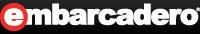Embarcadero Community JEDI Links
 How can I calculate a checksum by modulus 10 which is used in barcodes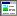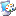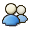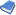03-Jun-03
 Category Algorithm Language Delphi 2.x Views 149 User Rating No Votes # Votes 0 Replies 0
 Publisher: DSP, Administrator Reference URL: DKB
 ``` Author: Mike Shkolnik How can I calculate a checksum by modulus 10 which is used in barcodes? Answer: Solve 1: I want to publish a code for checksum calculation by modulus 10 which is used in the barcodes. I must say that this "mod10" is specifical so readf an article if you're interested. This algorithm is very popular for UPC barcodes (Universal Product Code), hash code or serial number generation for applications etc... The basic algorithm: add the values of the digits in the odd positions (1, 3, 5...) multiply this result by 3 add the values of the digits in the even positions (2, 4, 6...) sum the results of steps 2 and 3 the check digit is the smallest number which, when added to the result in step 4, produces a multiple of 10. Small example. Assume the source data is 08137919805 0+1+7+1+8+5=22 22*3=66 8+3+9+9+0=29 66+29=95 95+??=100 where ?? is a 5 (our checksum) My implementation in the Pascal: 1 function Mod10(const Value: string): Integer; 2 var 3 i, intOdd, intEven: Integer; 4 begin 5 {add all odd seq numbers} 6 intOdd := 0; 7 i := 1; 8 while (i <= Length(Value)) do 9 begin 10 Inc(intOdd, StrToIntDef(Value[i], 0)); 11 Inc(i, 2); 12 end; 13 14 {add all even seq numbers} 15 intEven := 0; 16 i := 2; 17 while (i <= Length(Value)) do 18 begin 19 Inc(intEven, StrToIntDef(Value[i], 0)); 20 Inc(i, 2); 21 end; 22 23 Result := 3 * intOdd + intEven; 24 {modulus by 10 to get} 25 Result := Result mod 10; 26 if Result <> 0 then 27 Result := 10 - Result 28 end; You can expand or optimize this algorithm for own needs. For example, I modified it and now I use it for any characters (not only digits) in source value. The original algorithm I used for UPC-barcode validation in the SMReport Designer and the my extended algorithm I use in the serial number generation as part of the protection schema (in the shareware projects). Solve 2: 29 function BarCodeValid(ACode: string): boolean; 30 var 31 I: integer; 32 SumOdd, SumEven: integer; 33 ADigit, AChecksumDigit: integer; 34 begin 35 SumOdd := 0; 36 SumEven := 0; 37 for I := 1 to (Length(ACode) - 1) do 38 begin 39 ADigit := StrToIntDef(ACode[I], 0); 40 if (I mod 2 = 0) then 41 begin 42 SumEven := SumEven + ADigit; 43 end 44 else 45 begin 46 SumOdd := SumOdd + ADigit; 47 end; {if} 48 end; {for} 49 AChecksumDigit := StrToIntDef(ACode[Length(ACode)], 0); 50 Result := ((SumOdd * 3 + SumEven + AChecksumDigit) mod 10 = 0); 51 end; {--BarCodeValid--} ```